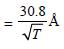Courses

# 28 Year NEET Questions: Dual Nature of Radiation and Matter- 1

## 35 Questions MCQ Test Physics 28 Years Past year papers for NEET/AIPMT Class 12 | 28 Year NEET Questions: Dual Nature of Radiation and Matter- 1

Description
This mock test of 28 Year NEET Questions: Dual Nature of Radiation and Matter- 1 for NEET helps you for every NEET entrance exam. This contains 35 Multiple Choice Questions for NEET 28 Year NEET Questions: Dual Nature of Radiation and Matter- 1 (mcq) to study with solutions a complete question bank. The solved questions answers in this 28 Year NEET Questions: Dual Nature of Radiation and Matter- 1 quiz give you a good mix of easy questions and tough questions. NEET students definitely take this 28 Year NEET Questions: Dual Nature of Radiation and Matter- 1 exercise for a better result in the exam. You can find other 28 Year NEET Questions: Dual Nature of Radiation and Matter- 1 extra questions, long questions & short questions for NEET on EduRev as well by searching above.
QUESTION: 1

### Which one of the following statements is nottrue for cathode rays?      

Solution:

Cathode rays are negatively charged rays.
Hence, when electric field is applied to them,
then they deflect in direction opposite to
electric field.

QUESTION: 2

### When ultraviolet radiation is incident on asurface, no photoelectrons are emitted. If asecond beam causes photoelectrons to beejected, it may consists of    

Solution:

Energy of photon of X-rays is more than
energy of photon of ultraviolet rays. Because
frequency of X rays is more than ultraviolet
rays.

QUESTION: 3

### A photoelectric cell is illuminated by a pointsource of light 1m away. When the source isshifted to 2m then 

Solution:

Power ∝ No. of electrons emitted (N)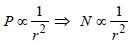QUESTION: 4

According to Einstein’s photoelectric equation, the graph between the kinetic energy of photoelectrons ejected and the frequency of
incident radiation is 

Solution:

K.E. = hν – φ
∴ graph of K.E. versus ν is a straight line
with +ve slope h and K.E. > 0 for ν such that
hν > φ.

QUESTION: 5

A photosensitive metallic surface has workfunction, hv0. If photons of energy 2 hv0 fallon this surface, the electrons come out with amaximum velocity of 4 ×106 m/s. When thephoton energy is increased to 5 hv0, thenmaximum velocity of photoelectrons will be



Solution:

We know that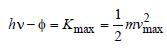According to question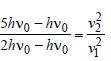v2 = 2v1 = 2 × 4× 106 = 8 × 106 m/s.

QUESTION: 6

The work functions for metals A, B and C arerespectively 1.92 eV, 2.0 eV and 5 eV.According to Einstein s equation, the metalswhich will emit photoelectrons for a radiationof wavelength 4100 Å is/are 

Solution: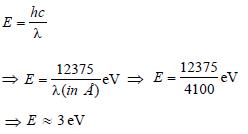So, only metals having work function less
than 3eV can emit photoelectrons for the
incident radiation of wavelength 4100Å.

QUESTION: 7

When photons of energy hv fall on an aluminiumplate (of work function E0), photoelectrons ofmaximum kinetic energy K are ejected. If thefrequency of the radiation is doubled, themaximum kinetic energy of the ejectedphotoelectrons will be     

Solution:

Applying Einstein's formula for photoelectricity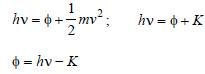If we use 2v frequency then let the kinetic
energy becomes K'
So,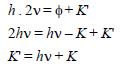QUESTION: 8

In a discharge tube ionization of enclosed gas isproduced due to collisions between 

Solution:

When electrons emitted from cathode collide
with gas molecules or atoms, they knock out
outer electrons and produce positively
charged ions. They become part of positive
rays.

QUESTION: 9

A photo-cell employs photoelectric effect toconvert      

Solution:

A photo-cell employs photoelectric effect to
convert light energy into photoelectric
current.

QUESTION: 10

The momentum of a photon of energy 1 MeV inkg m/s, will be 

Solution:

1 MeV = 106 × 1.6 × 10–19 joule
Momentum of photon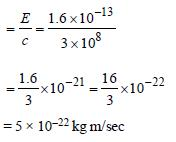QUESTION: 11

Monochromatic light of frequency 6.0 × 1014 Hzis produced by a laser. The power emitted is 2 × 10–3 ω. The number of photons emitted, onthe average, by the sources per second is            

Solution:

Since p = nhv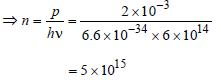QUESTION: 12

A 5 watt source emits monochromatic light ofwavelength 5000 Å. When placed 0.5 m away, itliberates photoelectrons from a photosensitivemetallic surface. When the source is moved to adistance of 1.0 m, the number of photoelectronsliberated will be reduced by a factor of 

Solution: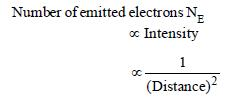Therefore, as distance is doubled, NE
decreases by (1/4) times.

QUESTION: 13

The work function of a surface of aphotosensitive material is 6.2 eV. The wavelengthof incident radiation for which the stoppingpotential is 5 V lies in the: 

Solution:

Work function, φ0 = 6.2eV.
Stopping potential V0 = 5V.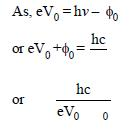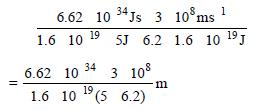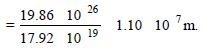Thus the wavelength of the incident radiation
lies in the ultraviolet region.

QUESTION: 14

In the phenomenon of electric discharge throughgases at low pressure, the coloured glow in thetube appears as a result of: 

Solution:

The coloured glow in the tube appears as a
result of excitations of electrons in the
atoms.

QUESTION: 15

A particle of mass 1 mg has the same wavelengthas an electron moving with a velocity of 3×10ms–1. The velocity of the particle is:    
(mass of electron = 9.1×10–31 kg)

Solution:

Wavelength of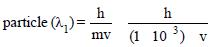where v is the velocity of the particle.
Wave length of electron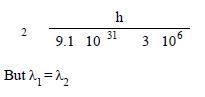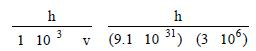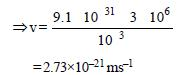QUESTION: 16

The number of photo electrons emitted for lightof a frequency v (higher than the threshold frequency v0) is proportional to: 

Solution:

The number of photoelectrons emitted is
proportional to the intensity of incident
light. Saturation current  ∝ intensity.

QUESTION: 17

The figure shows a plot of photo current versus anode potential for a photo sensitive surface for three different radiations. Which one of the following is a correct statement?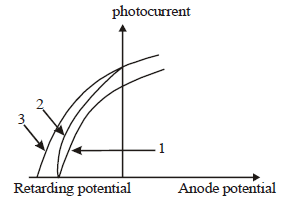Solution:

Retarding potential depends on the
frequency of incident radiation but is
independent of intensity.

QUESTION: 18

Monochromatic light of wavelength 667 nm isproduced by a helium neon laser. The poweremitted is 9 mW. The number of photons arrivingper sec on the average at a target irradiated bythis beam is: 

Solution:

λ = 667 × 10–9m, P = 9 ×10–3W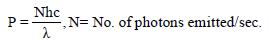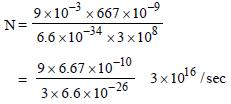QUESTION: 19

A beam of cathode rays is subjected to crossed Electric (E) and Magnetic fields (B). The fields are adjusted such that the beam is not deflected. The specific charge of the cathode rays is given by: 

Solution:

For no deflection of beam, eE = evB

⇒ v = E/B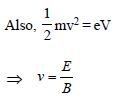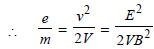QUESTION: 20

A source S1 is producing, 1015 photons persecond of wavelength 5000 Å. Another source S2 is producing 1.02×1015 photons per secondof wavelength 5100Å Then, (power of S2) (power of S1) is equal to : 

Solution:

Energy emitted/sec by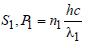Energy emitted/sec by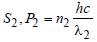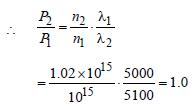QUESTION: 21

The potential difference that must be applied tostop the fastest photoelectrons emitted by anickel surface, having work function 5.01 eV,when ultraviolet light of 200 nm falls on it, mustbe:  

Solution: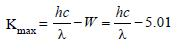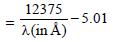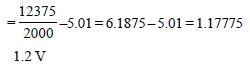QUESTION: 22

Photoelectric emmision occurs only whenthe incident light has more than a certainminimum 

Solution:

For occurence of photoelectric effect, the
incident light should have frequency more
than a certain minimum which is called the
threshold frequency (v0).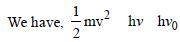For photoelectric effect emission v > v0
where v is the frequency of the incident
light.

QUESTION: 23

In the Davisson and Germer experiment, thevelocity of electrons emitted from the electrongun can be increased by 

Solution:

In the Davisson and Germer experiment, the
velocity of electrons emitted from the
electron gun can be increased by
increasing the potential difference between
the anode and filament.

QUESTION: 24

Light of two different frequencies whosephotons have energies 1 eV and 2.5 eVrespectively illuminate a metallic surface whosework function is 0.5 eV successively. Ratioof maximum speeds of emitted electrons will be

Solution:

The maximum kinetic energy of emitted
electrons is given by

K.E = φ – φ0
K.E1 = 1 eV – 0.5 eV = 0.5 eV
K.E2 = 2.5 eV – 0.5 eV = 2 eV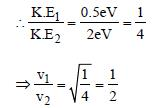QUESTION: 25

Electrons used in an electron microscope areaccelerated by a voltage of 25 kV. If the voltageis increased to 100kV then the de–Brogliewavelength associated with the electrons would

Solution: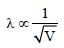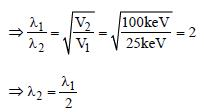QUESTION: 26

In photoelectric emission process from a metalof work function 1.8 eV, the kinetic energy ofmost energetic electrons is 0.5 eV. Thecorresponding stopping potential is     

Solution:

The stopping potential is equal to maximum
kinetic energy.

QUESTION: 27

The threshold frequency for a photosensitivemetal is 3.3 × 1014 Hz. If light of frequency 8.2 × 1014 Hz is incident on this metal, the cut-offvoltage for the photoelectric emission is nearly   [2011M]

Solution:

K.E. = hv – hvth = eV0 (V0 = cut off voltage)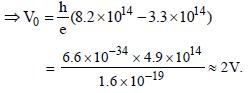QUESTION: 28

A 200 W sodium street lamp emits yellow lightof wavelength 0.6 μm. Assuming it to be 25%  efficient in converting electrical energy to light,the number of photons of yellow light it emitsper second is 

Solution:

Give that, only 25% of 200W converter
electrical energy into light of yellow colour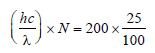Where N is the No. of photons emitted per
second, h = plank’s constant, c, speed of
light.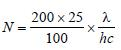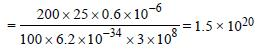QUESTION: 29

Monochromatic radiation emitted when electronon hydrogen atom jumps from first excited tothe ground state irradiates a photosensitivematerial. The stopping potential is measured tobe 3.57 V. The threshold frequency of the materialsis : 

Solution: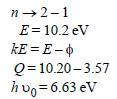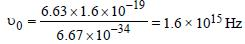QUESTION: 30

If the momentum of electron is changed by P,then the de Broglie wavelength associated withit changes by 0.5%. The initial momentum ofelectron will be : [2012M]

Solution:

The de-Broglie’s wavelength associated

with the moving electron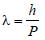Now, according to problem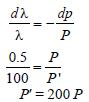QUESTION: 31

Two radiations of photons energies 1 eV and 2.5 eV, successively illuminate a photosensitivemetallic surface of work function 0.5 eV. The ratioof the maximum speeds of the emitted electronsis : [2012M]

Solution:

According to Einsten’s photoelectric effect,
the K.E. of the radiated electrons

K.Emax = E-W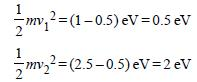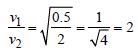QUESTION: 32

For photoelectric emission from certain metal the cut-off frequency is v. If radiation of frequency 2v impinges on the metal plate, the maximum possible velocity of the emitted electron will be (m is the electron mass) [NEET 2013

Solution:

From photo-electric equation,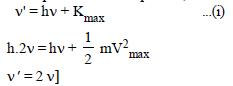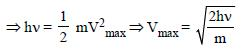QUESTION: 33

The wavelength λe of an electron and λp of a photon are of same energy E are related by   [NEET 2013]

Solution: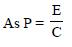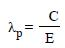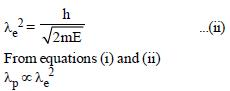QUESTION: 34

A source of light is placed at a distance of 50 cm from a photocell and the stopping potential is found to be V0. If the distance between the lightsource and photocell is made 25 cm, the newstopping potential will be [NEET Kar. 2013]

Solution:

Since, stopping potential is independent
of distance hence new stopping potential
will remain unchanged i.e., new stopping
potential = V0.

QUESTION: 35

The de-Broglie wavelength of neutron in thermal equilibrium at temperature T is       [NEET Kar. 2013]

Solution:

From formula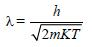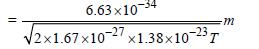[By placing value of h, m and k)